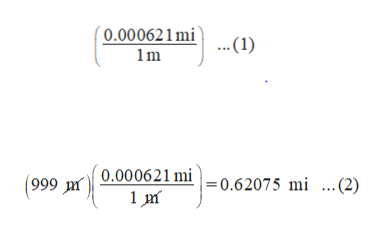how do i convert 999m to mi with the correct significant figures

Question

how do i convert 999m to mi with the correct significant figures

Step 1

The relation between m and mi is shown as follows:

1 m = 0.000621 mi

The conversion factor used to convert m into mi is shown by the expression as shown in equation (1).

Multiply the conversion factor with 999 m as shown in equation (2).help_outlineImage Transcriptionclose( 0.000621mi ... (1) 1m 999 0.000621 mi = 0.62075 mi ...(2) fullscreen
Step 2

Since the given value contains three significant figures, thus, the final answer wil...

Want to see the full answer?

See Solution

Want to see this answer and more?

Our solutions are written by experts, many with advanced degrees, and available 24/7

See Solution
Tagged in

Chemistry